# Resources tagged with: Addition & subtraction

Filter by: Content type:
Age range:
Challenge level:

### There are 216 results### Take Three Numbers

##### Age 7 to 11 Challenge Level:

What happens when you add three numbers together? Will your answer be odd or even? How do you know?### Consecutive Negative Numbers

##### Age 11 to 14 Challenge Level:

Do you notice anything about the solutions when you add and/or subtract consecutive negative numbers?### Number Pyramids

##### Age 11 to 14 Challenge Level:

Try entering different sets of numbers in the number pyramids. How does the total at the top change?### Oddly

##### Age 7 to 11 Challenge Level:

Find the sum of all three-digit numbers each of whose digits is odd.### Cayley

##### Age 11 to 14 Challenge Level:

The letters in the following addition sum represent the digits 1 ... 9. If A=3 and D=2, what number is represented by "CAYLEY"?### Two and Two

##### Age 11 to 16 Challenge Level:

How many solutions can you find to this sum? Each of the different letters stands for a different number.### Number Daisy

##### Age 11 to 14 Challenge Level:

Can you find six numbers to go in the Daisy from which you can make all the numbers from 1 to a number bigger than 25?### Aba

##### Age 11 to 14 Challenge Level:

In the following sum the letters A, B, C, D, E and F stand for six distinct digits. Find all the ways of replacing the letters with digits so that the arithmetic is correct.### Tis Unique

##### Age 11 to 14 Challenge Level:

This addition sum uses all ten digits 0, 1, 2...9 exactly once. Find the sum and show that the one you give is the only possibility.### Eleven

##### Age 11 to 14 Challenge Level:

Replace each letter with a digit to make this addition correct.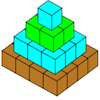### Open Squares

##### Age 7 to 11 Challenge Level:

This task, written for the National Young Mathematicians' Award 2016, focuses on 'open squares'. What would the next five open squares look like?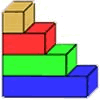### Rod Measures

##### Age 7 to 11 Challenge Level:

Using 3 rods of integer lengths, none longer than 10 units and not using any rod more than once, you can measure all the lengths in whole units from 1 to 10 units. How many ways can you do this?### Diagonal in a Spiral

##### Age 7 to 11 Challenge Level:

Investigate the totals you get when adding numbers on the diagonal of this pattern in threes.### Sheep Talk

##### Age 7 to 11 Challenge Level:

In sheep talk the only letters used are B and A. A sequence of words is formed by following certain rules. What do you notice when you count the letters in each word?##### Age 11 to 14 Challenge Level:

Make a set of numbers that use all the digits from 1 to 9, once and once only. Add them up. The result is divisible by 9. Add each of the digits in the new number. What is their sum? Now try some. . . .### Football Sum

##### Age 11 to 14 Challenge Level:

Find the values of the nine letters in the sum: FOOT + BALL = GAME### Number Differences

##### Age 7 to 11 Challenge Level:

Place the numbers from 1 to 9 in the squares below so that the difference between joined squares is odd. How many different ways can you do this?### Cows and Sheep

##### Age 7 to 11 Challenge Level:

Use your logical reasoning to work out how many cows and how many sheep there are in each field.### Pole Star Sudoku 2

##### Age 11 to 16 Challenge Level:

This Sudoku, based on differences. Using the one clue number can you find the solution?### Magic Vs

##### Age 7 to 11 Challenge Level:

Can you put the numbers 1-5 in the V shape so that both 'arms' have the same total?### Making Maths: Double-sided Magic Square

##### Age 7 to 14 Challenge Level:

Make your own double-sided magic square. But can you complete both sides once you've made the pieces?### Countdown

##### Age 7 to 14 Challenge Level:

Here is a chance to play a version of the classic Countdown Game.##### Age 11 to 14 Challenge Level:

If you take a three by three square on a 1-10 addition square and multiply the diagonally opposite numbers together, what is the difference between these products. Why?### Got it for Two

##### Age 7 to 14 Challenge Level:

Got It game for an adult and child. How can you play so that you know you will always win?### Subtraction Surprise

##### Age 7 to 14 Challenge Level:

Try out some calculations. Are you surprised by the results?### Charitable Pennies

##### Age 7 to 14 Challenge Level:

Investigate the different ways that fifteen schools could have given money in a charity fundraiser.### An Introduction to Magic Squares

##### Age 7 to 16##### Age 5 to 11 Challenge Level:

Try out this number trick. What happens with different starting numbers? What do you notice?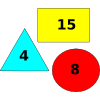### Super Value Shapes

##### Age 7 to 11 Challenge Level:

If each of these three shapes has a value, can you find the totals of the combinations? Perhaps you can use the shapes to make the given totals?### Have You Got It?

##### Age 11 to 14 Challenge Level:

Can you explain the strategy for winning this game with any target?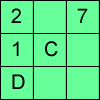### Alphabetti Sudoku

##### Age 11 to 16 Challenge Level:

This Sudoku requires you to do some working backwards before working forwards.### Sticky Dice

##### Age 7 to 11 Challenge Level:

Throughout these challenges, the touching faces of any adjacent dice must have the same number. Can you find a way of making the total on the top come to each number from 11 to 18 inclusive?### Making Pathways

##### Age 7 to 11 Challenge Level:

Can you find different ways of creating paths using these paving slabs?### Calendar Capers

##### Age 11 to 14 Challenge Level:

Choose any three by three square of dates on a calendar page...### Got it Article

##### Age 7 to 14

This article gives you a few ideas for understanding the Got It! game and how you might find a winning strategy.### Number Tracks

##### Age 7 to 11 Challenge Level:

Ben’s class were cutting up number tracks. First they cut them into twos and added up the numbers on each piece. What patterns could they see?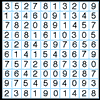### A Numbered Route

##### Age 7 to 11 Challenge Level:

Can you draw a continuous line through 16 numbers on this grid so that the total of the numbers you pass through is as high as possible?### Odds and Threes

##### Age 7 to 11 Challenge Level:

A game for 2 people using a pack of cards Turn over 2 cards and try to make an odd number or a multiple of 3.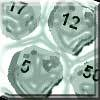### Asteroid Blast

##### Age 7 to 11 Challenge Level:

A game for 2 people. Use your skills of addition, subtraction, multiplication and division to blast the asteroids.### Adding and Subtracting Positive and Negative Numbers

##### Age 11 to 14

How can we help students make sense of addition and subtraction of negative numbers?### Zargon Glasses

##### Age 7 to 11 Challenge Level:

Zumf makes spectacles for the residents of the planet Zargon, who have either 3 eyes or 4 eyes. How many lenses will Zumf need to make all the different orders for 9 families?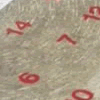### All Seated

##### Age 7 to 11 Challenge Level:

Look carefully at the numbers. What do you notice? Can you make another square using the numbers 1 to 16, that displays the same properties?### Weights

##### Age 11 to 14 Challenge Level:

Different combinations of the weights available allow you to make different totals. Which totals can you make?### X Is 5 Squares

##### Age 7 to 11 Challenge Level:

Can you arrange 5 different digits (from 0 - 9) in the cross in the way described?### One Million to Seven

##### Age 7 to 11 Challenge Level:

Start by putting one million (1 000 000) into the display of your calculator. Can you reduce this to 7 using just the 7 key and add, subtract, multiply, divide and equals as many times as you like?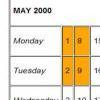### Calendar Patterns

##### Age 7 to 11 Challenge Level:

In this section from a calendar, put a square box around the 1st, 2nd, 8th and 9th. Add all the pairs of numbers. What do you notice about the answers?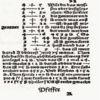### Mathematical Symbols

##### Age 5 to 14

A brief article written for pupils about mathematical symbols.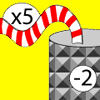### Function Machines

##### Age 7 to 11 Challenge Level:

If the numbers 5, 7 and 4 go into this function machine, what numbers will come out?### The Money Maze

##### Age 7 to 11 Challenge Level:

Go through the maze, collecting and losing your money as you go. Which route gives you the highest return? And the lowest?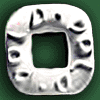### Polo Square

##### Age 7 to 11 Challenge Level:

Arrange eight of the numbers between 1 and 9 in the Polo Square below so that each side adds to the same total.